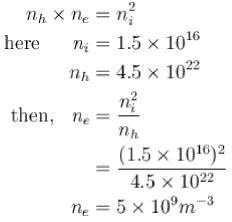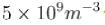Courses

# Semiconductor Diode MCQ Level – 1

## 10 Questions MCQ Test Topic wise Tests for IIT JAM Physics | Semiconductor Diode MCQ Level – 1

Description
This mock test of Semiconductor Diode MCQ Level – 1 for Physics helps you for every Physics entrance exam. This contains 10 Multiple Choice Questions for Physics Semiconductor Diode MCQ Level – 1 (mcq) to study with solutions a complete question bank. The solved questions answers in this Semiconductor Diode MCQ Level – 1 quiz give you a good mix of easy questions and tough questions. Physics students definitely take this Semiconductor Diode MCQ Level – 1 exercise for a better result in the exam. You can find other Semiconductor Diode MCQ Level – 1 extra questions, long questions & short questions for Physics on EduRev as well by searching above.
QUESTION: 1

### The correct sequence of band gaps of germanium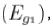silicon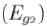and gallium arsenide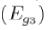will :

Solution:

The energy gap of gallium is maximum whereas silicon has less Eg than that of germanium.
The correct answer is: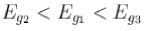QUESTION: 2

### In the circuit having an ideal diode, shown below, the rms voltage across R is.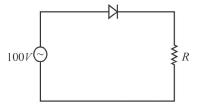Solution:

When the diode is forward biased, it behaves like a closed switch and connects the a.c. supply to the load RL. However, when the diode is reverse biased, it behaves like an open switch and disconnects the a.c. supply from the load RL. This switching action of diode permits only the positive half-cycles of input a.c. voltage to appear across RL.
In ac. circuit, the potential is given in terms of rms hence,
Here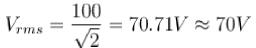The correct answer is: 70 V

QUESTION: 3

### A zener diode is also a p-n junction diode. It can be used as :

Solution:

A Zener diode is used to get constant DC voltage from a DC unregulated output of a rectifier.

QUESTION: 4

The electrical conductivity of a semi-conductor in cases when electromagnetic radiation of wave length shorter than 2480 nm is incident on it. What is the band - gap Mark -0 .33 out of 1.00 (in eV) for the semiconductor?
(take h = 6.67 x10-34 J-s).

Solution: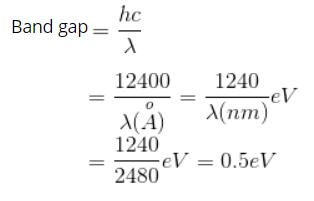QUESTION: 5

If in a p-n junction diode, a square input signal of 8V is applied (see figure below), what is the value of output signal across RL?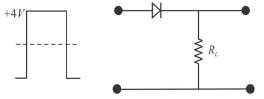Solution:

This is a general case of half-wave rectifier. For positive signal, the diode will be in ON state and signal passes through it but for negative half of signal, diode is reverse biased and it goes in non-conducting state and signal is blocked.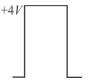QUESTION: 6

The impurity atoms with which pure silicon should be doped to make p-type semiconductor are those of .

Solution:

When an element of fifth group is doped in pure germanium/silicon due to excess of an electron, the semiconductor is known as n-type semiconductor. In n-type semiconductor, majority of carrier is electron. If the element of third group is added, the semiconductor is known as p-type. In this case, majority of carriers is hole.
The correct answer is: boron and aluminium

QUESTION: 7

Which one of the following circuits is to be selected for doubling the input voltage?

Solution:

The two diodes are chosen for doubling the input voltage.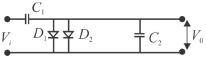QUESTION: 8

The fermi level of an intrinsic semi-conductor is pinned at the centre of the band-gap. The probability of occupation of the highest electronic state in valence band at room temperature, will b e :

Solution:

According to Fermi-Dirac distribution law, the probability of an electron in finding energy level E at temperature T is given as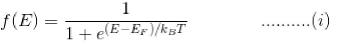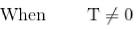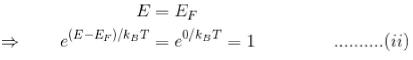By (i) and (ii),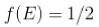QUESTION: 9

The depletion layers in a p-n junction diode consists of layers of :

Solution:

The depletion layer in p-n junction consists of the layer of positively charged donors on the n-side and negatively charged acceptors on p-side.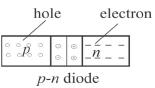The correct answer is: Positively charged donors on the n-side and negatively charged acceptors on the p-side

QUESTION: 10

pure silicon at 300K has equal electrons concentrations (ne) and hole concentration (nh) of 1.5 x 1016 m-3 . Doping by sodium increases nto 4.5 x 1022 m-3 . Then ne in the doped silicon is :

Solution:

Under thermal equilibrium, the product of free electron concentration n and hole 9 e concentration nh is constant equal to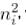where ni is the concentration of intrinsic semiconductor